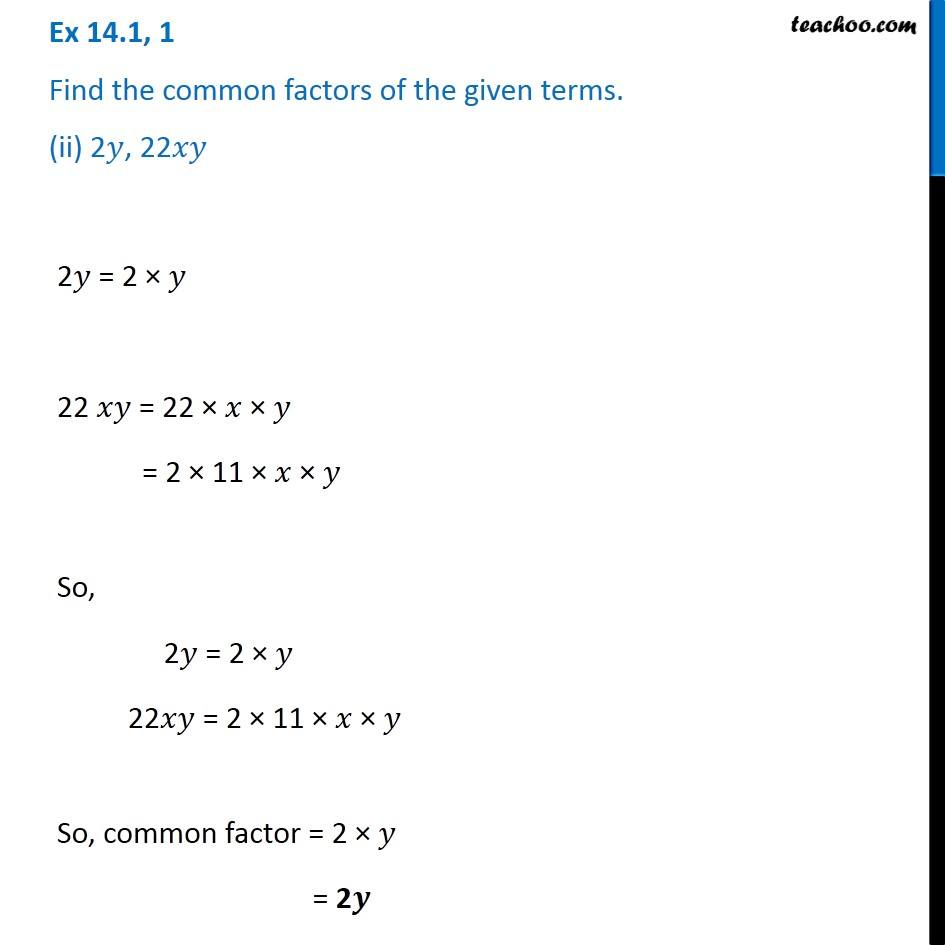Factorisation using common factors

Chapter 12 Class 8 Factorisation
Concept wiseLearn in your speed, with individual attention - Teachoo Maths 1-on-1 Class

### Transcript

Ex 12.1, 1 Find the common factors of the given terms. (iii) 14 𝑝𝑞, 〖28𝑝〗^2 𝑞^2 14𝑝𝑞 = 14 × 𝑝 × 𝑞 = 2 × 7 × 𝑝 × 𝑞 28 𝑝^2 𝑞^2 = 28 × 𝑝^2 × 𝑞^2 = 2 × 2 × 7 × 𝑝^2 × 𝑞^2 = 2 × 2 × 7 × 𝑝 × 𝑝 "× " 𝑞" × " 𝑞 So, 14𝑝𝑞 = 2 × 7 × 𝑝 × 𝑞 28 𝑝^2 𝑞^2 = 2 × 2 × 7 × 𝑝 × 𝑝 "× " 𝑞" × " 𝑞